## Posts Tagged ‘kisei’

### Kisei 2011- Cho U Equals the Score in Game 2

Saturday, January 29th, 2011

After losing the first game to challenger Iyama Yuta, Kisei title holder Cho U won the second game.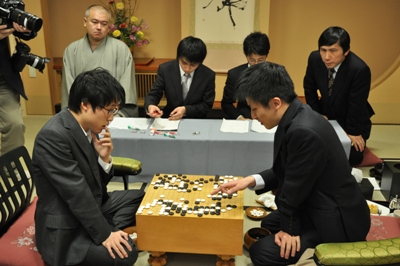I found Iyama’s move 40 quite amazing, it is played in a place that would never occur to me, see the next diagram.In the game black replied by connecting with the triangle marked stone. This makes move 40 a very efficient forcing move, since now white has even more forcing moves from the outside. This diagram shows the strongest continuation for white – in fact white did play this, but much later in the game.The last diagram shows why black cannot reply aggressively in response to white 40.Here is the complete game.

(; EV[35th Kisei title match 2] KM[6.5] FF SZ GM PW[Iyama Yuta] WR[Meijin] DT[2011-01-26,27] BR[Kisei] RE[B+R] PB[Cho U] ;B[qd];W[dc];B[pq];W[cp];B[nc];W[po];B[qm];W[qq];B[mq];W[pp];B[pr] ;W[ro];B[eq];W[do];B[pj];W[qr];B[ip];W[ci];B[fd];W[hd];B[dd];W[ed] ;B[ee];W[ec];B[cc];W[fe];B[cd];W[ff];B[ef];W[fc];B[cg];W[eg];B[dg] ;W[eh];B[eo];W[en];B[fo];W[dq];B[er];W[be];B[df];W[jp];B[jo];W[kp] ;B[mo];W[io];B[ho];W[in];B[iq];W[kn];B[hn];W[im];B[dn];W[cn];B[dm] ;W[cm];B[dl];W[cl];B[ph];W[ld];B[me];W[qc];B[pc];W[le];B[mf];W[lf] ;B[lg];W[md];B[mh];W[nd];B[od];W[rd];B[rc];W[ob];B[qb];W[ng];B[nh] ;W[kg];B[qn];W[mn];B[qo];W[qp];B[no];W[nn];B[lp];W[ko];B[kr];W[mg] ;B[lh];W[og];B[pg];W[oe];B[oc];W[pf];B[qf];W[qe];B[re];W[pe];B[rg] ;W[kh];B[lb];W[bg];B[bh];W[ah];B[bf];W[af];B[ag];W[rf];B[sf];W[bg] ;B[bi];W[cf];B[ch];W[ce];B[bd];W[de];B[ad];W[ae];B[ai];W[jr];B[jq] ;W[cb];B[ag];W[kq];B[lr];W[ah];B[bb];W[ac];B[bc];W[ba];B[ab];W[oj] ;B[ok];W[db];B[ag];W[pk];B[pl];W[ah];B[dk];W[ca];B[ag];W[nk];B[qk] ;W[ah];B[bo];W[aa];B[co];W[bk];B[ck];W[bj];B[cj];W[di];B[bm];W[bl] ;B[bn];W[dh];B[ag];W[ac];B[bf];W[mb];B[al];W[mc];B[nb];W[na];B[rn] ;W[mj];B[oi];W[gl];B[fk];W[gk];B[on];W[oq];B[or];W[nr];B[nq];W[op] ;B[ns];W[ps];B[mr];W[sp];B[qs];W[rr];B[sn];W[sq];B[fi];W[gh];B[lj] ;W[lk];B[ki])

### Kisei 2011 – Iyama Yuta Wins Game 1

Friday, January 14th, 2011

Iyama Yuta who challenges Cho U for the Japanese Kisei title won game one by resignation.Here is the exchange that took place at the end of the game:The continuation is puzzling though – did Cho U make a mistake, or just set the scene for resignation? Here are the final moves. After black cuts with 6, a and b are miai for black, and he wins the semeai on the upper side.White should have played as in the following diagram. The game looks close to me after this – in fact white looks a little ahead, but maybe I am missing something.Here is the complete game.

(;FFGMSZSTCA[UTF-8]AP[SGFC:1.16] EV[Kisei 2011, game 1] PB[Iyama Yuta] BR[9DP] HA PW[Cho U] WR[9DP] KM[6.5] DT[2011-01-14] RE[B+R] ;B[pd];W[dp];B[qp];W[ed];B[cd];W[cc];B[ic];W[dd];B[ce];W[bc] ;B[cn];W[fp];B[dj];W[oq];B[kq];W[on];B[qm];W[iq];B[ko];W[ol] ;B[pk];W[ok];B[pj];W[oj];B[pi];W[jp];B[kp];W[km];B[jo];W[im] ;B[ho];W[gq];B[hr];W[ir];B[hm];W[in];B[io];W[hn];B[hq];W[gr] ;B[fo];W[hp];B[gn];W[dn];B[dm];W[en];B[il];W[jl];B[jm];W[jn] ;B[kn];W[jm];B[lm];W[ik];B[lk];W[mn];B[ln];W[hl];B[gm];W[fl] ;B[po];W[oo];B[pq];W[pr];B[or];W[nr];B[gp];W[go];B[op];W[nq] ;B[gp];W[hs];B[ep];W[go];B[np];W[mp];B[gp];W[fq];B[eo];W[do] ;B[eq];W[go];B[er];W[dl];B[cq];W[lr];B[kr];W[js];B[mq];W[no] ;B[pp];W[lq];B[lp];W[mo];B[gp];W[cm];B[ls];W[mr];B[go];W[ks] ;B[dg];W[dq];B[dr];W[bq];B[cp];W[co];B[bp];W[bo];B[br];W[gc] ;B[nc];W[ci];B[di];W[cj];B[ch];W[eh];B[ck];W[bk];B[eg];W[fh] ;B[fg];W[gh];B[gg];W[hg];B[hf];W[if];B[he];W[ig];B[ie];W[qd] ;B[qe];W[pc];B[od];W[rc];B[re];W[ob];B[nb];W[qb];B[qr];W[dh] ;B[bh];W[bj];B[hj];W[hi];B[gl];W[ij];B[gk];W[fj];B[rd];W[qc] ;B[hb];W[gb];B[jf];W[jg];B[kf];W[mg];B[kg];W[kh];B[ps];W[pl] ;B[ql];W[pn];B[qn];W[me];B[og];W[ng];B[lh];W[oh];B[ki];W[of] ;B[jh];W[ii];B[pg];W[oi];B[ph];W[ld];B[lc];W[hc];B[ib];W[kc] ;B[kb];W[jd];B[jc];W[kd];B[id];W[mc];B[fk];W[ek];B[lb];W[ae] ;B[fm];W[bf];B[em];W[dk];B[fd];W[fe];B[gd];W[ee];B[ge];W[cf] ;B[eb];W[fc];B[ec];W[db];B[ea];W[ga];B[dc])

### Kisei 2011 Started

Thursday, January 13th, 2011

The most important Japanese title in professional Go, Kisei, started yesterday.

The title holder is Cho U, while the challenger is Iyama Yuta who seems to be in very good shape recently (he defeated Cho U in the final of the Daiwa Cup).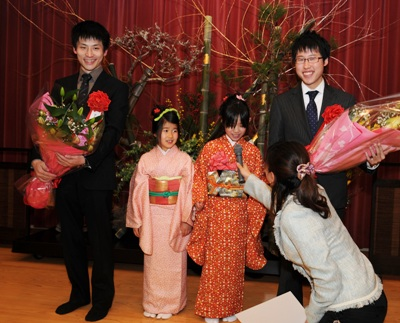Here is the game at the end of day one – Cho U plays white and he made the sealed move. White’s shape in the center looks bad, like damezumari, but he can capture some black stones and fix that.### Cho U Captures Kisei Title!

Thursday, February 25th, 2010

Game five of the 34th Kisei title resulted in Cho U’s win, which ensured his capturing of the title from Yamashita Keigo by a 4-1 score. Yamashita captured the Kisei title in 2006 from Hane Naoki, then defended it for 3 consecutive years.

It looks like Cho U’s bad form from 2009 has not followed him in 2010.Here is the game record from the last game: Cho U played white and won by 1.5 points.

(;CA[Windows-1252]SZAP[MultiGo:4.4.4]GN[34th Kisei Title, game 5]EV[34th Kisei Title] DT[2010-02-26]PB[Yamashita Keigo]BR[9p]BT[Japan]PW[Cho U]WR[9p]WT[Japan]KM[6.5] HARE[W+1.5]MULTIGOGM ;B[qd];W[pp];B[dd];W[dp];B[pj];W[nd];B[oc];W[jd];B[od];W[fc];B[cf];W[qm];B[hc];W[hd] ;B[id];W[ie];B[ic];W[gd];B[jc];W[kd];B[lb];W[jf];B[cn];W[fp];B[dk];W[dg];B[fg];W[df] ;B[cg];W[de];B[ce];W[ed];B[dh];W[dc];B[cd];W[jq];B[ee];W[fe];B[ef];W[qe];B[pe];W[qf] ;B[ph];W[rd];B[qc];W[rc];B[rg];W[qg];B[qh];W[rf];B[pq];W[qq];B[oq];W[qr];B[no];W[dn] ;B[cq];W[co];B[fr];W[dr];B[bo];W[dq];B[cm];W[jo];B[lq];W[on];B[jr];W[ir];B[kr];W[hq] ;B[pg];W[op];B[np];W[pr];B[or];W[nn];B[mn];W[lo];B[mo];W[pf];B[of];W[rh];B[ri];W[sg] ;B[rl];W[rm];B[ip];W[jp];B[is];W[hs];B[hm];W[js];B[jm];W[km];B[nm];W[om];B[kn];W[jn] ;B[jl];W[ln];B[mm];W[kl];B[kk];W[lk];B[lj];W[mk];B[kj];W[nf];B[ng];W[mg];B[ne];W[mf] ;B[me];W[nh];B[og];W[le];B[ll];W[lm];B[ml];W[md];B[oe];W[lc];B[mb];W[nk];B[lp];W[ko] ;B[ol];W[mi];B[lh];W[li];B[ki];W[kh];B[jh];W[kg];B[cr];W[el];B[dl];W[gl];B[hl];W[gk] ;B[hi];W[mr];B[mq];W[os];B[ns];W[nr];B[nq];W[ps];B[nl];W[ql];B[ok];W[cc];B[eb];W[ec] ;B[em];W[fn];B[ek];W[gm];B[ho];W[hn];B[in];W[gn];B[io];W[iq];B[ds];W[hk];B[ik];W[im] ;B[il];W[kb];B[kc];W[hb];B[gb];W[ib];B[jb];W[gc];B[ka];W[fb];B[er];W[ij];B[hj];W[bd] ;B[be];W[qj];B[qi];W[bc];B[rb];W[sb];B[go];W[fo];B[gj];W[cp];B[bp];W[gg];B[gh];W[ff] ;B[eg];W[hg];B[qk];W[rk];B[rj];W[sl];B[ii];W[qb];B[pb];W[ra];B[en];W[gp];B[eo];W[fq] ;B[jj];W[gr];B[jg];W[ig];B[lg];W[kf];B[nj];W[mj];B[ni];W[mh];B[oh];W[lf];B[pm];W[pn] ;B[pl];W[fk];B[fj];W[do];B[si];W[sk];B[sh];W[rg];B[oo];W[po];B[ia];W[ga];B[pa];W[fl] ;B[dm];W[nc];B[nb];W[ae];B[af];W[ad];B[qa];W[rb];B[ih])

More photos here. You can also see a couple of videos from the previous game here.

### Yamashita’s First Win in Kisei Title Defense

Friday, February 19th, 2010

Yamashita Keigo won for the first time in this year’s Kisei title match, trying to defend the title against Cho U. The score is now 3-1 for Cho U, who has 3 more chances to capture the title from Yamashita.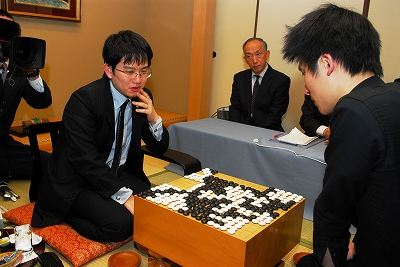Here is the record of the forth game. Yamashita Keigo, playing white, won by 7.5 points.

(;GMFFCA[UTF-8]AP[CGoban:3]ST RU[Japanese]SZKM[6.50] GN[Kisei Title 2010, game 4]PW[Yamashita Keigo]PB[Cho U]WR[9p]BR[9p]DT[2010-02-19]EV[Kisei Title 2010]RE[W+7.50]MULTIGOGM ;B[pd] ;W[dp] ;B[qp] ;W[dd] ;B[fq] ;W[cn] ;B[lq] (;W[pm] ;B[pk] ;W[op] (;B[qn] ;W[mp]LB[qm:A][pn:B]C[Since A and B are miai for black, white decided to change the course and possibly decide between A and B later, depending on black's response on the lower side.] ;B[lp] ;W[lo] ;B[mq] (;W[qq] (;B[rq] ;W[ko] (;B[iq] (;W[pq] ;B[pn] ;W[rr] ;B[om] ;W[nc] ;B[np] ;W[no] ;B[mo] ;W[mn] ;B[oo] ;W[mp] ;B[nq] ;W[rp] ;B[ro] ;W[sq] ;B[lc] ;W[ld] ;B[kd] ;W[md] ;B[jc] ;W[pf] ;B[of] ;W[qc] ;B[qd] ;W[pc] ;B[od] ;W[oc] ;B[pg] ;W[gc] ;B[kf] ;W[ce] ;B[mf] ;W[lk] ;B[dm] ;W[cm] ;B[dk] ;W[gp] ;B[dq] ;W[cq] ;B[cp] ;W[do] ;B[cr] ;W[bq] ;B[er] ;W[br] ;B[dh] ;W[lg] ;B[lf] ;W[je] ;B[he] ;W[hd] ;B[ge] ;W[ke] ;B[ie] ;W[id] ;B[jd] ;W[jf] ;B[fc] ;W[gd] ;B[fd] ;W[fb] ;B[jg] ;W[ig] ;B[fg] ;W[ih] ;B[ec] ;W[hb] ;B[dc] ;W[cc] ;B[cb] ;W[ff] ;B[gf] ;W[jb] ;B[bc] ;W[bd] ;B[cd] ;W[hq] ;B[ip] ;W[ir] ;B[jr] ;W[gr] ;B[is] ;W[hr] ;B[eo] ;W[go] ;B[fo] ;W[hn] ;B[in] ;W[im] ;B[jn] ;W[jm] ;B[kn] ;W[km] ;B[jp] ;W[fm] ;B[fn] ;W[gm] ;B[dn] ;W[co] ;B[cl] ;W[cc] ;B[bm] ;W[df] ;B[fe] ;W[ep] ;B[fp] ;W[eq] ;B[if] ;W[le] ;B[kg] ;W[ch] ;B[ci] ;W[bi] ;B[di] ;W[bg] ;B[bj] ;W[dg] ;B[ai] ;W[cd] ;B[lm] ;W[gh] ;B[fh] ;W[el] ;B[dl] ;W[nj] ;B[nl] ;W[ll] ;B[ji] ;W[ki] ;B[kj] ;W[lj] ;B[li] ;W[mi] ;B[kh] ;W[ij] ;B[oi] ;W[fi] ;B[fj] ;W[mm] ;B[ln] ;W[ml] ;B[hl] ;W[hk] ;B[il] ;W[jl] ;B[kk] ;W[kl] ;B[gk] ;W[gl] ;B[ik] ;W[jk] ;B[hj] ;W[hm] ;B[hk] ;W[fr] ;B[bo] ;W[bp] ;B[bn] ;W[dr] ;B[rc] ;W[rb] ;B[rd] ;W[ii] ;B[gi] ;W[hi] ;B[ei] ;W[fk] ;B[gj] ;W[jj] ;B[mh] ;W[or] ;B[mo] ;W[ej] ;B[fi] ;W[mp] ;B[nr] ;W[ni] ;B[nh] ;W[kp] ;B[kq] ;W[pp] ;B[mo] ;W[oj] ;B[pj] ;W[mp] ;B[ic] ;W[ib] ;B[mo] ;W[pi] ;B[oh] ;W[mp] ;B[oq] ;W[so] ;B[sn] ;W[pr] ;B[mo] ;W[qo] ;B[rn] ;W[mp] ;B[nd] ;W[mc] ;B[mo] ;W[ef] ;B[ab] ;W[ba] ;B[gg] ;W[mp] ;B[bb] ;W[mo] ;B[lr] ;W[eb] ;B[db] ;W[bh] ;B[sb] ;W[ra] ;B[ek] ;W[ah] ;B[aj] ;W[nk] ;B[bf] ;W[ad] ;B[af] ;W[ee] ;B[cf] ;W[cg] ;B[ed] ;W[ol] ;B[pl] ;W[nm] ;B[on] ;W[be] ;B[ns] ;W[ki] ;B[kj] ;W[ao] ;B[eg] ;W[ae] ;B[os] ;W[hs] ;B[js] ;W[me] ;B[ne] ;W[an] ;B[bl] ;W[da] ;B[ca] ;W[ea] ;B[ps] ;W[qs] ;B[am] ;W[ap] ;B[hg] ;W[hh] ;B[ok] ;W[po] ;B[fl] ;W[em] ;B[en] ;W[nl] ;B[fk] ;W[sp]) (;W[qm] ;B[pn] (;W[om] ;B[on] ;W[nm] ;B[nn] ;W[mn] ;B[no]) (;W[on] ;B[om] ;W[ol] ;B[nm] ;W[pl] ;B[nn])) (;W[nc] ;B[pf] ;W[jd] ;B[on] ;W[mo] ;B[om])) (;B[pn] ;W[on] (;B[np] ;W[no] ;B[mo] ;W[mn] ;B[oq] ;W[mp] ;B[nq] ;W[qm]) (;B[om] ;W[jq] ;B[np] ;W[mo] ;B[oq] ;W[no] ;B[nq] ;W[ol] ;B[nm] ;W[pl])) (;B[on] ;W[pn] ;B[po] ;W[oo] ;B[om] ;W[jq] ;B[pq] ;W[oq] ;B[qr] ;W[or] ;B[jr] ;W[ir] ;B[iq] ;W[ip] ;B[hq] ;W[kr] ;B[jp] ;W[kq] ;B[hr] ;W[kp] ;B[dr])) (;B[ko] (;W[pp] ;B[ro] ;W[pn] ;B[ql] ;W[kn] ;B[jo] ;W[jn] ;B[io] ;W[mo]) (;W[qm]))) (;W[mo]) (;W[ko] (;B[np] ;W[no] ;B[mo] ;W[mn] (;B[oq] ;W[mp] ;B[nq] ;W[qm]) (;B[pn] (;W[on] ;B[oq] ;W[mp] ;B[nq]) (;W[nq] ;B[mp] ;W[on]))) (;B[pn] ;W[on] ;B[np] ;W[oq]))) (;B[oo] ;W[no] ;B[po] ;W[nn] ;B[np] ;W[mp] ;B[nq] ;W[mq]LB[lq:A]C[Black's stone A would be hurt badly this way.])) (;W[ip]C[Also possible.]))

Next game will be played on February 25th and 26th. You can find the complete tournament table here.

### Cho U Leads 3-0 in Kisei Title

Friday, February 5th, 2010

Cho U seems to be back in good shape after a bad year: he is just one win away from capturing the Kisei title from Yamashita Keigo.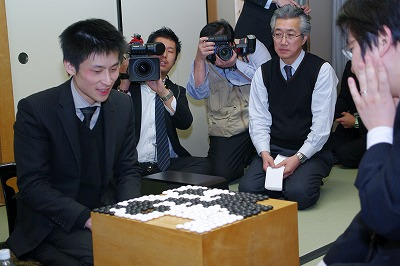Here is the record of the third game. Cho U, white, won by 8.5 points after a very confusing multi-step ko fight involving a large black group.

(;GMFFCA[UTF-8]AP[CGoban:3]ST RU[Japanese]SZKM[6.50] PW[Cho U]PB[Yamashita Keigo]WR[9p]BR[9p]DT[2010-02-03,04]EV[34th Kisei title match 3]RE[W+8.50] ;B[pd] ;W[dp] (;B[qp] (;W[ed] (;B[op] ;W[cd] (;B[pj] (;W[nc] ;B[pf] (;W[kd] ;B[dj] (;W[cl] (;B[jp] (;W[ek] (;B[fi] (;W[hj] ;B[df] ;W[ej] ;B[ei] ;W[di] ;B[dh] ;W[ci] ;B[fe] ;W[fd] ;B[ge] ;W[gd] ;B[he] ;W[hd] ;B[gk] ;W[hk] ;B[gl] ;W[hl] ;B[el] ;W[dk] ;B[gj] ;W[hn] ;B[ch] ;W[bi] ;B[je] ;W[jd] ;B[fp] ;W[hp] ;B[en] ;W[lp] ;B[ko] ;W[np] ;B[nq] ;W[oo] ;B[pp] ;W[mq] ;B[no] ;W[mo] ;B[mp] ;W[gm] ;B[fm] ;W[np] ;B[nn] ;W[nr] ;B[mp] ;W[gi] ;B[np] ;W[fj] ;B[gh] ;W[hi] ;B[cn] ;W[bp] ;B[dq] ;W[cq] ;B[eq] ;W[kq] ;B[co] ;W[cp] ;B[gn] ;W[hm] ;B[dr] ;W[cr] ;B[go] ;W[ho] ;B[bo] ;W[gp] ;B[gq] ;W[fr] ;B[fq] ;W[hq] ;B[gr] ;W[fo] ;B[eo] ;W[fn] ;B[ep] ;W[bm] ;B[ao] ;W[lo] ;B[mr] ;W[lr] ;B[lq] ;W[br] ;B[aq] ;W[mq] ;B[ce] ;W[bd] ;B[lq] ;W[hg] ;B[gg] ;W[hh] ;B[ke] ;W[le] ;B[lf] ;W[mf] ;B[lg] ;W[mg] ;B[ie] ;W[lh] ;B[kh] ;W[me] ;B[oc] ;W[nb] ;B[mq] ;W[qh] ;B[qj] ;W[pg] ;B[nd] ;W[qf] ;B[qe] ;W[rf] ;B[lc] ;W[lb] ;B[md] ;W[kc] ;B[of] ;W[ld] ;B[re] ;W[pb] ;B[qc] ;W[ne] ;B[kp] ;W[qb] ;B[rb] ;W[ra] ;B[oe] ;W[rc] ;B[rd] ;W[sb] ;B[pc] ;W[hr] ;B[jr] ;W[ap] ;B[cs] ;W[bs] ;B[ar] ;W[ds] ;B[gs] ;W[jq] ;B[kr] ;W[hs] ;B[es] ;W[dm] ;B[em] ;W[fl] ;B[jg] ;W[nh] ;B[mk] ;W[cm] ;B[cs] ;W[gf] ;B[eh] ;W[ds] ;B[do] ;W[bn] ;B[cs] ;W[nj] ;B[nk] ;W[ds] ;B[bq] ;W[an] ;B[cs] ;W[oj] ;B[ok] ;W[ds] ;B[fk] ;W[fl] ;B[cs] ;W[fg] ;B[fh] ;W[ds] ;B[fk] ;W[gk] ;B[cs] ;W[rj] ;B[rk] ;W[ds] ;B[dd] ;W[dl] ;B[cs] ;W[ee] ;B[ef] ;W[ds] ;B[go] ;W[gn] ;B[cs] ;W[hf] ;B[ff] ;W[ds] ;B[dc] ;W[dn] ;B[cs] ;W[as] ;B[aq] ;W[er] ;B[bq] ;W[ds] ;B[fs] ;W[er] ;B[cs] ;W[ar] ;B[id] ;W[ic] ;B[hc] ;W[hb] ;B[gc] ;W[fc] ;B[bq] ;W[ds] ;B[gb] ;W[fr] ;B[fb] ;W[be] ;B[bf] ;W[ae] ;B[cc] ;W[bc] ;B[bb] ;W[af] ;B[bg] ;W[ag] ;B[ah] ;W[bh] ;B[ac] ;W[ai] ;B[pi] ;W[ri] ;B[og] ;W[ph] ;B[jl] ;W[sk] ;B[rl] ;W[ib] ;B[iq] ;W[jn] ;B[kn] ;W[jm] ;B[kl] ;W[eb] ;B[ec] ;W[ga] ;B[de] ;W[fa] ;B[db] ;W[ea] ;B[sl] ;W[oh] ;B[li] ;W[km] ;B[lm] ;W[mc] ;B[mh] ;W[sf] ;B[ij] ;W[ji] ;B[jj] ;W[ii] ;B[ob] ;W[oa] ;B[ki] ;W[il] ;B[sj] ;W[od] ;B[nd] ;W[mj] ;B[lj] ;W[jk] ;B[kk] ;W[ik] ;B[si] ;W[sh] ;B[sk] ;W[ng] ;B[da] ;W[ha] ;B[qi] ;W[rh] ;B[kj] ;W[if] ;B[jf] ;W[js] ;B[ks] ;W[ip] ;B[ir] ;W[jo] ;B[oi] ;W[ni] ;B[is] ;W[mi] ;B[lh]) (;W[dh] (;B[di] ;W[eh] (;B[fk] ;W[fl] ;B[gk] ;W[gl] ;B[hk] ;W[hl] ;B[ik] ;W[jm]) (;B[fj] ;W[gl]) (;B[dk] ;W[dl] ;B[el] ;W[fk] ;B[fj] ;W[em] ;B[fl] ;W[gk])) (;B[ej] ;W[fk])) (;W[gk] ;B[hi] ;W[dh] ;B[ej]) (;W[gj] ;B[gi] ;W[hj] ;B[hi] ;W[ij] ;B[jh] ;W[kj] ;B[id])) (;B[dg] ;W[ef] ;B[fi]) (;B[ej] ;W[fk] ;B[fj] ;W[gk])) (;W[fq]) (;W[dh] ;B[ek] ;W[dn])) (;B[dg] ;W[jp]) (;B[ek] ;W[jq])) (;W[jq] ;B[cn] ;W[fp])) (;W[pb]) (;W[jp]) (;W[jc])) (;W[dj]) (;W[cj])) (;B[cn] ;W[ck])) (;B[cd] (;W[cc]) (;W[df])) (;B[cc])) (;W[dc] ;B[fq] ;W[cn] ;B[lq] ;W[de] ;B[pj] ;W[nd] ;B[lc] ;W[me] ;B[qf]) (;W[dd] ;B[op]) (;W[op])) (;B[pq] ;W[dd] ;B[fq] ;W[ip] ;B[dn] ;W[fp] ;B[cq] ;W[eq] ;B[cp] ;W[cl] ;B[bn] ;W[gq]))

Next game will be played on February 18th and 19th.

The complete tournament table is here.

### Kisei Title Started: Cho U Won Game One

Friday, January 15th, 2010

Cho U, challenger in this year’s Kisei title match, won game one against title holder Yamashita Keigo.

In their most recent encounter, Yamashita captured the Tengen title from Cho U last month.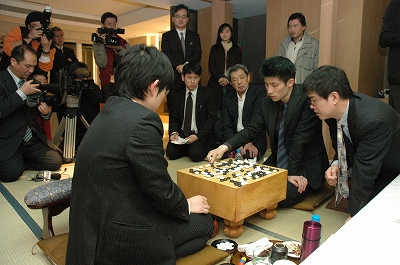The record of game one is here. Cho U, playing white, won by resignation.

(;GMFFCA[UTF-8]AP[CGoban:3]ST RU[Japanese]SZKM[6.50] GN[34th Kisei Title Match, game 1]PW[Cho U]PB[Yamashita Keigo]WR[9p]BR[9p]DT[2010-01-14,15]EV[34th Kisei Title Match]RE[W+Resign]MULTIGOGM ;B[qd] ;W[pp] ;B[dd] (;W[dp] (;B[qn] (;W[pk] (;B[np] ;W[pn] (;B[qq] (;W[qo] ;B[pq] (;W[lq] (;B[nr] (;W[qm] (;B[fq] (;W[cn] (;B[dr] (;W[gq] (;B[gp] ;W[hq] (;B[hp] (;W[fr] (;B[eq] (;W[ip] (;B[fo] (;W[ck] (;B[oc] (;W[mq] (;B[iq] ;W[ir] ;B[jq] ;W[jp] ;B[nq] ;W[jr] ;B[cq] ;W[rp] ;B[rq] ;W[cp] ;B[er] ;W[fc] ;B[hc] ;W[df] ;B[ec] ;W[fd] ;B[ee] ;W[eb] ;B[db] ;W[ed] ;B[de] ;W[dc] ;B[cc] ;W[ge] ;B[jd] ;W[qf] ;B[oe] ;W[cf] ;B[ef] ;W[dh] ;B[eh] ;W[di] ;B[ec] ;W[gg] ;B[pg] ;W[rd] ;B[rc] ;W[dc] ;B[ei] ;W[gi] ;B[ej] ;W[pd] ;B[qc] ;W[qh] ;B[dk] ;W[cl] ;B[ig] ;W[hg] ;B[eg] ;W[if] ;B[jf] ;W[jg] ;B[ih] ;W[je] ;B[ie] ;W[kf] ;B[hf] ;W[jf] ;B[he] ;W[kd] ;B[gf] ;W[jc] ;B[id] ;W[hb] ;B[ic] ;W[ib] ;B[kc] ;W[jb] ;B[ld] ;W[ke] ;B[lc] ;W[gc] ;B[fg] ;W[jh] ;B[ii] ;W[ji] ;B[jj] ;W[kj] ;B[ij] ;W[kk] ;B[li] ;W[ki] ;B[qg] ;W[rg] ;B[rh] ;W[rf] ;B[ec] ;W[ga] ;B[ri] ;W[pf] ;B[pi] ;W[qj] ;B[qi] ;W[og] ;B[ph] ;W[of] ;B[ni] ;W[od] ;B[nd] ;W[ne] ;B[pc] ;W[oh] ;B[ok] ;W[oi] ;B[pj] ;W[oj] (;B[qk] ;W[pl] ;B[rl] ;W[md] ;B[nc] ;W[il] ;B[me] ;W[nf] ;B[nn] ;W[nm] ;B[jm] ;W[hk] ;B[cj] ;W[bi] ;B[jl] ;W[jk] ;B[ik] ;W[im] ;B[in] ;W[jn] ;B[kn] ;W[jo] ;B[mm] ;W[nl] ;B[ll] ;W[hh] ;B[fk] ;W[gl] ;B[bj] ;W[dj] ;B[ci] ;W[ch] ;B[bh] ;W[dl] ;B[ek] ;W[bk] ;B[ai] ;W[mo] ;B[no] ;W[mn] ;B[mp] ;W[lo] ;B[bq] ;W[bp] ;B[ap] ;W[ao] ;B[aq] ;W[bg] ;B[dg] ;W[cg] ;B[ce] ;W[af] ;B[ah] ;W[al] ;B[bo] ;W[aj] ;B[ak] ;W[sq] ;B[sr] ;W[aj] ;B[om] ;W[ol] ;B[ak] ;W[rk] ;B[rj] ;W[aj] ;B[ea] ;W[fb] ;B[ak] ;W[sp] ;B[lp] ;W[ko] ;B[rr] ;W[aj] ;B[hm] ;W[hl] ;B[ak] ;W[an] ;B[bn] ;W[aj] ;B[kq] ;W[kp] ;B[ak] ;W[am] ;B[bm] ;W[aj] ;B[kr] ;W[lr] ;B[ak] ;W[bl] ;B[em] ;W[aj] ;B[ks] ;W[ls] ;B[ak] ;W[dn] ;B[eo] ;W[aj] ;B[da] ;W[bi]C[Black resigned.]) (;B[pl] ;W[qk] ;B[ql] ;W[ol] ;B[rk] ;W[nk] ;B[rj])) (;B[nq] ;W[cq]) (;B[cq])) (;W[cq] ;B[iq] ;W[ir] ;B[jq] ;W[jr] ;B[jp] ;W[io] ;B[jo] ;W[in] ;B[jn] ;W[im] ;B[kr])) (;B[iq] ;W[ir] ;B[jq] ;W[jr] ;B[jp] ;W[mq] (;B[io] ;W[nq] ;B[oq] ;W[oc]) (;B[nq] ;W[io] ;B[jo] ;W[in] ;B[lo] ;W[ls])) (;B[jp] (;W[io] ;B[iq] ;W[ir] ;B[jq] ;W[jr] ;B[jo] ;W[in] ;B[jn] ;W[im] ;B[kr]) (;W[iq] ;B[io] ;W[jo] ;B[kp] ;W[kr] ;B[jn] ;W[ko] ;B[lp]))) (;W[io] ;B[cq] ;W[ck]) (;W[mq] ;B[nq] ;W[cq] ;B[iq] ;W[ir] ;B[jq] ;W[jr] ;B[kq] ;W[kr] ;B[io] (;W[jp] ;B[kp] ;W[jo] ;B[lp] ;W[in] ;B[mp] ;W[ho] ;B[fm] ;W[dk] ;B[lr] ;W[er] ;B[dq] ;W[gs] ;B[hm] ;W[jl]) (;W[ck]))) (;B[cl] ;W[dl] ;B[dk] ;W[dm] (;B[fo] ;W[ck] ;B[cj] ;W[bk] ;B[dj] ;W[bl] ;B[kc]) (;B[cq] ;W[fp] ;B[ek] ;W[er] ;B[dq] ;W[fo])) (;B[iq] ;W[ir] ;B[jq] ;W[jr] ;B[kq] (;W[kr] ;B[io] ;W[jp] ;B[kp] ;W[jo] ;B[lp] ;W[fp]) (;W[io] ;B[kr] ;W[fp] ;B[hn] ;W[ep])) (;B[io] ;W[jp] ;B[fo] ;W[ck])) (;W[iq] ;B[cq] ;W[ck])) (;B[iq] ;W[ir] ;B[jr] ;W[ip] ;B[jq] ;W[eq] ;B[fp] ;W[er])) (;W[iq])) (;B[fp] ;W[cq]) (;B[cq] ;W[fr] ;B[eq] ;W[hp] ;B[fo] ;W[ck]) (;B[fr] ;W[cq] ;B[hp] ;W[iq] ;B[ep] (;W[jo]) (;W[oc] ;B[jo])) (;B[ep] ;W[dq] ;B[cr] (;W[bq] ;B[eq] ;W[hp] ;B[gn] ;W[ck]) (;W[eo] ;B[eq] ;W[fo] ;B[cp] ;W[do] ;B[bo]))) (;B[gr] ;W[fr] (;B[gp] ;W[hq] ;B[hr] ;W[eq] ;B[fp] ;W[er] ;B[iq]) (;B[er] ;W[fp] ;B[fs] ;W[hq]))) (;W[cq] ;B[iq]) (;W[hq] ;B[cq] ;W[eq] ;B[er] ;W[fp] ;B[gp] ;W[gq] ;B[fr] ;W[go] ;B[ci])) (;B[iq]LB[dr:A])) (;W[hp]) (;W[eq] ;B[fp] ;W[dn] ;B[ip] ;W[ko])) (;B[oc] (;W[fq] ;B[cf]) (;W[cf] ;B[fc] ;W[bd])) (;B[lc])) (;W[rp]) (;W[od] (;B[oc] ;W[pd] ;B[pc] ;W[qe] ;B[nc] ;W[rd] ;B[qc] ;W[pf] ;B[qm] ;W[pm] ;B[ro] ;W[ql] ;B[qp]) (;B[pf] ;W[qc] ;B[rc] ;W[pc] ;B[re] ;W[lc] ;B[qm] ;W[pm] ;B[ql]))) (;B[mq] ;W[lp] ;B[oc] ;W[no]) (;B[qm] (;W[pm] ;B[ro] ;W[ql] ;B[qp]C[Too much to expect for black.]) (;W[rp] ;B[pm] ;W[on] ;B[om] ;W[nn] ;B[nm] ;W[mn] ;B[mq] ;W[lp] ;B[rq] ;W[nk]))) (;W[od] ;B[oc] ;W[pd] ;B[pc] ;W[qe] ;B[nc] (;W[rd] ;B[qc] ;W[pf]) (;W[nd] ;B[md] ;W[qc] ;B[rd] ;W[qb] ;B[rc])) (;W[qm] ;B[oc] (;W[nq] ;B[oq] ;W[mq] ;B[op] ;W[mp]) (;W[oq] ;B[nq]) (;W[lq] ;B[mq] ;W[lp]))) (;W[pq] ;B[pm] ;W[po] ;B[pl] (;W[ok] ;B[nm] ;W[rp]) (;W[qk] (;B[ro] ;W[rq] ;B[nm] ;W[qg]) (;B[ph])))) (;B[pm] ;W[on] ;B[qo] ;W[op] ;B[rq] ;W[om])) (;B[on] ;W[np] ;B[pi]) (;B[qq] ;W[qp] ;B[pq] ;W[op] ;B[rp] ;W[ro] ;B[rq] ;W[qo] ;B[nq]) (;B[pi] ;W[np] ;B[kd]) (;B[fq] ;W[cn] ;B[np] ;W[pn] ;B[pm] ;W[on] ;B[qp] ;W[qo] ;B[ro] ;W[po] ;B[rm] ;W[qq] ;B[rp] ;W[om] ;B[jq])) (;W[nq] (;B[pj]) (;B[kd] (;W[qo] ;B[pn] ;W[pj] ;B[ph] ;W[nj] ;B[mn]) (;W[pf] (;B[od] ;W[qe] ;B[rd] ;W[qj]) (;B[ph] ;W[pd] ;B[pe] ;W[oe] ;B[qe] ;W[qc] ;B[of] ;W[pc] ;B[qf] ;W[nf] ;B[pg] ;W[nd]))) (;B[od])) (;W[np])) (;B[kc])) (;W[cp] ;B[ep] ;W[gq] ;B[cq] ;W[cm] ;B[dn] ;W[bo] ;B[fq] ;W[gp] ;B[bq] ;W[cj] ;B[oc] ;W[qn] ;B[pj] ;W[fc] ;B[hc] ;W[df] ;B[fd] ;W[cc] ;B[cd] ;W[dc] ;B[ed] ;W[fb] ;B[gc] ;W[bc] ;B[nf] ;W[gd]))

The tournament table is here. Game two will be played on January 27 and 28.

### Cho U Kisei Challenger

Monday, November 16th, 2009

Cho U defeated O Rissei in the playoff to decide the Kisei challenger. The Kisei title holder is Yamashita Keigo, who is currently challenging Cho U in Tengen title.The game record is here.

(; GM KM[6.5] SZ FF WR[Judan] EV[34th Kisei challenger decision match] DT[2009-11-16] PB[O Rissei] RE[W+R] PW[Cho U] BR[9d] ;B[pd];W[dd];B[qp];W[op];B[dp];W[qq];B[kq];W[rp];B[qo];W[ro];B[pq] ;W[pp];B[qn];W[qr];B[pj];W[nc];B[qf];W[pb];B[cf];W[fc];B[di];W[fq] ;B[eq];W[fp];B[dn];W[ip];B[ko];W[jq];B[mq];W[qm];B[on];W[pm];B[hq] ;W[hp];B[fr];W[gr];B[er];W[qc];B[gm];W[pn];B[me];W[im];B[mc];W[md] ;B[ld];W[nd];B[ne];W[lc];B[kd];W[co];B[do];W[cn];B[cp];W[ck];B[cj] ;W[bj];B[bi];W[dj];B[ci];W[bl];B[mb];W[nb];B[aj];W[bp];B[bq];W[dm] ;B[em];W[dl];B[ej];W[el];B[fl];W[ao];B[bk];W[aq];B[ar];W[br];B[ap] ;W[en];B[bo];W[fm];B[fn];W[em];B[gl];W[eo];B[km];W[jl];B[cc];W[cd] ;B[dc];W[ec];B[bd];W[be];B[bc];W[ce];B[bf];W[ic];B[gd];W[fe];B[ge] ;W[ae];B[db];W[eb];B[ba];W[jb];B[id];W[kc];B[kj];W[fj];B[fk];W[ek] ;B[ei];W[jj];B[ji];W[gj];B[hk];W[ij];B[hj];W[ii];B[hi];W[ki];B[hc] ;W[hb];B[jd];W[jc];B[jh];W[kk];B[lj];W[ih];B[lk];W[jg];B[kh];W[kg] ;B[lh];W[hg];B[if];W[ig];B[lg];W[bn];B[an];W[rj];B[rk];W[qk];B[qj] ;W[gh];B[gi];W[fg];B[fh];W[eh];B[fi];W[ri];B[ql];W[pk];B[pl];W[ok] ;B[ol];W[rl];B[rm];W[om];B[sl];W[nl];B[rl];W[nk];B[po];W[nm];B[rn] ;W[oo];B[qh];W[rh];B[io];W[hn];B[ho];W[gn];B[nn];W[np])

### Yamashita Wins Game 6, Defends Kisei Title 4:2

Thursday, March 12th, 2009
Yamashita Keigo defended his Kisei title against challenger Yoda Norimoto by 4:2.Yamashita, playing white, won the 6th game by 2.5p after building a lot of thickness in the center early on, which translated into a big moyo.Game record for game 6 is here.